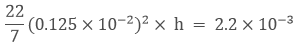Newbie

# 2.2 cubic dm of brass is to be drawn into a cylindrical wire of 0.25 cm in diameter. Find the length of the wire?

• 0

This question is of chapter 16, exercise 16.1 mensuration from rd sharma.

In this question we have to find length of the wire. Please someone provide me solution.

Share

1. 2.2 dm3 of brass is to be drawn into a cylindrical wire of Diameter = 0.25 cm

So, radius of the wire (r) = d/2

= 0.25/2 = 0.125*10-2 cm

Now, 1 cm = 0.01 m

So, 0.1cm = 0.001 m

Let the length of the wire be (h)

We know that,

Volume of the cylinder = πr2h

It’s understood that,

Volume of cylindrical wire = Volume of brass of 2.2 dm3h = 448 m

Therefore, the length of the cylindrical wire drawn is 448 m

• 0